# Warlock’s Tower 100% Walkthrough and Achievement Guide

06.11.2020

For Warlock’s Tower players, this is a guide of 100% walkthrough and complete achievement of the game, all you need are listed below, let’s check it out.### Dungeon A

Stage 1: The Beginning
R R R R┇R UStage 2: Every Gem Counts
U U U L┇L L L L┇D D D R┇L L U

Stage 3: Super Gem is Here
D D D R┇R D U U┇R R D R┇U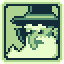Talked to the Warlock
Talk to the Evil Warlock for the first time

Stage 4: Shortcuts Are Evil
L L L L┇L D D D┇R R R R┇R D

Stage 5: No Be Greedy, Tim
L L D L┇L L L U┇U U L U

Stage 6: Dungeon Creeper
R R U R┇R U D D┇D R D

Stage 7: Gems Vs. Gems
L L L L┇L U U R┇D U U U┇R U

Stage 8: Tim, Don’t Be Fooled!
R R U U┇L L L L┇D D L L┇L U U U┇U

### Dungeon B

Stage 1: The Mail Always Gets Through
L L L L┇L U U U┇R R L L┇L L UStage 2: GEMinie Room
R R R D┇D D L R┇U R R U┇L R U R┇R U

Stage 3: Big Room Is Big
U U L L┇D L D U┇L L L U┇L R U U┇R R R R┇U R L L┇U L U

Stage 4: Don’t Get Gemmed Now
U U L U┇U U L L┇L D D D┇D R L L┇L L L U┇U R R L┇U U R U

Stage 5: So Close No Matter How Far
L D L R┇D R L D┇D D L R┇R D R R┇R R R U┇U U U L┇L D L R┇U U U U

Stage 6: The Walking Tim
D D L R┇R R U D┇R D D R┇R U U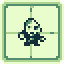Met the Zombie
Play a stage where there’s a Zombie

Stage 7: Zombie Is As Zombie Does
R R U R┇R U D R┇U L L U┇U L L U

Stage 8: A Key Concern
R R R R┇R U U U┇L L L L┇L U

Stage 9: Tim of the Dead
Achievement: U L D U┇U U U U┇U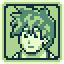Talked to the Hero
Talk to Lenda do Herói’s Hero

Solution
U L D U┇U U R R┇R U R R┇D L R U┇L U U U┇R R L L┇L L UCompleted the Dungeon
Beat all Dungeon stages

### The Factory A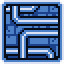Reached the Factory
Reach the game’s second worldStage 1: Doors Wide Shut
L L L D┇D D U L┇L L D R┇U U U U

Stage 2: Tim, the locksmith
R R D R┇R U D R┇R D D L┇L L L L┇L L U U┇R R R U┇U

Stage 3: Breaking Out
U U L R┇R R R D┇D R R U┇L U R U┇UGot the Magic Flag
Get the Magic Flag artifact

Stage 4: Jaaaaailbreak
D L D D┇D D D R┇R R D R┇D R R U┇L L L U┇U U U R┇D U U R┇R D U U┇R U

Stage 5: Tag Tim
D L L L┇C L L U┇UTalked to Jess
Talk to Jess for the first time

Stage 6: A Pair of Two
C R U R┇C R R U┇U U C R┇U U U C┇U D L L┇U U

Stage 7: Moving Together
C L C U┇R C L L┇L L L D┇C R R R┇R D R U┇U

Stage 8: The Key to Success
R R C U┇L L U U┇C R R U┇C L C U┇R U R U┇U U

Stage 9: Zombietween Us
R R C L┇D D D L┇L C U C┇L U U U┇R U

### The Factory B

Stage 1: Moving Grounds
R R U U┇L R D L┇L L U R┇R R UStage 2:Let it slide
D D D L┇U D U L┇L D D D┇D D R D

Stage 3: Getting Carried Around
D D L L┇D R R R┇U U U

Stage 4: Hello Darkness
U R R U┇U U L L┇U

D R L L┇D D D D┇D L R U┇R R R R┇R R U U┇U U L L┇U D R D┇D D R R┇U

Level 6: Whispers in the Dark.
Achievement:
D D R L┇D D D R┇L D D D┇R R R R┇U D U L┇U U U U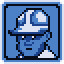Talked to Todd Torial
Talk to Puzzle Depot’s Todd Torial

Solution:
D D R L┇D D D R┇L D D D┇R R R R┇U R R R┇R D R U┇R D U U┇U U U

Stage 7: Gears of War(lock)
U U L U┇U L U R┇D D D U┇U L R L┇L U U

U U D L┇U U U R┇D U U U┇U U R R┇R R U D┇D D R R┇R U

Stage 9: Landsliding Victory
U R R L┇U U R U┇R U R R┇D U L U┇U U L L┇U U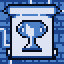Completed the Factory
Beat all Factory stages

### The Warehouse A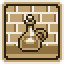Reached the Warehouse
Reach the game’s third worldStage 1: A Little Leverage
U R U U┇L U D L┇L U U D┇D D D L┇L L U R┇U U

Stage 2: At the Gates
R R R R┇U L L U┇L L L U┇R U U U┇U L R R┇U U U D┇L L R R┇U R D D┇R R U

Stage 3: The Mailman’s Dilemma
R D U R┇R R U L┇L D L L┇D D D L┇U R R R┇R R L U┇U U U U

Stage 4: A Slime in the Way
D L L R┇ R R R D┇ D L L DMet the Slime
Play a stage where there’s a Slime

Stage 5: Three of Acids
L R R R┇ U U U L ┇L L U U┇ R U

Stage 6: The Siege
L D L R ┇D D D R┇ R D D D┇ L L L L┇ L L L L ┇R U U U ┇L U U U┇ U R U

R R R D┇D D C D┇L C L L┇U C R C┇U U C D┇C U R U

Stage 8: They See Me Rolling
U U U R┇U U L U┇U D D R┇U R D R┇D D R U┇R R U

Stage 9: Pulling a Trick
C D D D┇L U C D┇R D D C┇U C R R┇D C L L┇U U U U┇C R U D┇D D D

### The Warehouse B

Stage 1: Jarring Situation
L L L L┇L L D D┇D D R R┇R R R D┇D DStage 2: WhisKEY in the Jar
R D D D┇L L L L┇U U U L┇L D D D┇L R R L┇U U U U

Stage 3: E-Vase-ive Action
L L D L┇L L U U┇L U U R┇R R U R┇U L D D┇D R D R┇R R U U┇U U U U

Stage 4: A Pressing Matter
U U D R┇R U R D┇L D D R┇U R R R┇R R U U┇U

Stage 5: Push Them Over
R R U R┇U L U L┇L U L U┇U R U

Stage 6: The Belly of the beast
R R R U┇U U D D┇D D R D┇R R L U┇U R U R┇U

Achievement:
D D L R┇R D R R┇R R D U┇UTalked to Racketboy
Talk to the Racketboy himself

Solution:
D D L R┇R D R L┇D D R R┇R D R R┇U R L L┇L L L U┇L L D L┇L D

Level 8: Under Pressure
U R R U┇U C U U┇C U R C┇U R R R┇C U C U┇U R R U

Stage 9: Evil is hatching
D D D D┇C U C U┇U R C L┇D L L L┇C R D R┇U U U U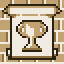Completed the Warehouse
Beat all Warehouse stages

### Library AReached the Library
Reach the game’s fourth worldStage 1: A New Technologics
U R U L┇U D D U┇U L L D┇D D D U┇L L L D┇U U U U┇U

Stage 2: Bending Space
U R R L┇R R R U┇L D R R┇R D U U┇R

Stage 3: Holesome
C R C U┇U U L L┇U U R R┇L L L C┇L L C L┇L U

D L L D┇L L D R┇L U D L┇D D D R┇D D L R┇R U U U┇U U

Stage 5: Fear of the Warp
U U U R┇U U D R┇L U R R┇L D R D┇R L D D┇R U R D┇U U R L┇U U U R┇U U U U

Stage 6: Eye in the sky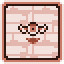Met the Fleye
Play a stage where there’s a Fleye

Note: After pressing L once and the conversation is over press R to restart the stage and begin the solution.
L L L L┇L U U R┇R U U U┇L L D R┇D U U U┇R U L L┇L L U

Stage 7: Locked in Sight
U U R R┇U L U D┇R R L U┇D D D L┇D L U D┇R R U R┇R D R U┇U U U U

Stage 8: Dropping Like Fleyes
U U U R┇R U R R┇U R D D┇D D L L┇U U U D┇L L U L┇L D D R┇R D R U┇U U R U┇U L U

Stage 9: What Goes Around
R R U R┇U D L L┇L L U U┇R D R R┇R U D D┇L R U L┇D L R U┇L R D L┇D R R U┇L U U U┇U R U

### Library B

Stage 1: A Tale of Two Jars
R R R U┇R U R R┇D R R R┇U D L D┇R R R R┇R L L R┇U U R U┇D R R U┇UStage 2: Freedom Hurts
U U R U┇L L U L┇L D L L┇L R R U┇U U L L┇U L L U┇R R L U┇U U U

Stage 3: The Middleman is Dead
U U L L┇D D R R┇R R U D┇R U R L┇U R U U┇D D L U┇R R L L┇L D D D┇U U R U┇L U L U┇D D L L┇U U U

Stage 4: Guardians of the Lost Gem
C L R L┇R R R C┇R U L U┇U R L U┇L R R U┇R U

Stage 5: Serial Manuevers
R R L R┇R R U L┇U R R L┇L U D L┇L L U D┇R D L U┇R R U

Stage 6: Lost Within Books
U U R R┇U R U U┇L D R U┇U U D L┇R R R L┇U L U R┇R U R U┇D R U L┇L L U

Stage 7: Of Monsters and Machines
D D D D┇D R C R┇R D D D┇C L C D┇L C L L┇U R L L┇C L D C┇U U R U┇U U

Stage 8: The Lever’s Dance
Achievement:
R D L U┇R R R D┇R R U D┇R L L U┇D L D D┇D D D R┇R L L R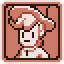Talked to Rubick
Talk to Möira’s Rubick

Sulution:
R D L U┇R R R D┇R R U D┇R L L U┇D L D D┇D D D R┇R L L L┇U U D D┇D R D

Stage 9: For Each a Pair
D C U D┇R C U C┇R R C R┇R C R C┇R C U U┇C R R C┇D R R C┇R C U D┇L U R C┇R U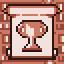Completed the Library
Beat all Library stages

### Chambers A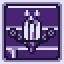Reached the Chambers
Reach the game’s last worldStage 1: Hole in my Soul
L L U U┇L U L U┇U D R R┇R U R D┇R R U U┇R D R R┇U U L L┇L L U

Stage 2: A Fair Matchup
U L L C┇L C R U┇C U U U┇C U U U┇L U D C┇R C D D┇C R C D┇C R U

Stage 3: Intricate Machinery
U U L L┇D U L U┇U L R R┇U D R L┇R D R R┇R L U U┇U

Stage 4: A Monsterous Effort
R R L L┇L U U L┇U R R R┇R U U U┇R D D U┇L R U L┇R R L L┇U L R D┇R R R D

Stage 5: Stepping on Toes
U R C R┇C R C R┇L C U C┇U C L L┇C U R C┇D C R R┇U U C D┇L C D L┇R R C L┇C R U U┇D D R R┇U U U

Stage 6: Next to Impossibru!
U R R D┇R U D D┇U R R U┇U U D D┇R R U U┇R R U U┇R U L D┇U L U L┇L L U

Stage 7: Zombiepocalypse
C D C U┇U R C U┇R R C L┇C R R C┇R D U C┇R R C L┇C R R C┇R C R R┇C L C R┇U U U L┇L L U U┇R R D R┇U U

Stage 8: A Well Shut Way
R R R L┇D R R U┇U D D D┇L U U L┇L R U R┇U L R R┇R D R U┇R U U U┇U R
L L┇R R R R┇D L D D┇D U

Stage 9: The Open/Closed Dillema
L U R R┇L L L R┇R D L L┇U U L R┇R R L D┇D L D R┇U U U R┇U R R U

### Chambers B

Stage 1: Hopscotch
R C L D┇C R R D┇R D D D┇D DStage 2: The Hall of Pain
R U L R┇R R R U┇L L L L┇U L L L┇D D L R┇U R R U┇L L U R┇R D R L┇L R L R┇R U R U┇U U U

Stage 3: Do the convolution
Achievement:
C D L C┇L D L C┇RD R L ┇C D L L┇D U U U┇U C D L┇D R R L┇U R C R┇U U C R┇L R R C┇L U C R┇R U C U┇D R R C┇U U L UTalked to Kim
Talk to Keen’s Kim

Solution:
C D L C┇L D L C┇R D R L┇C D L L┇D U U U┇U C D L┇D R R L┇U R C R┇U U C R┇L R R C┇L U C R┇R U C U┇D R R C┇U U R U┇U

Stage 4: Critter Gang
U R R U┇U R U U┇U L U U┇U R U U

Stage 5: Around The World
U U D D┇R R U R┇R R L U┇U U UL┇D L U L┇R D D D┇R D R R┇R D R U┇U U U R┇R U U L┇L D R U┇U

Stage 6: Archipelago
L D U L┇ R R L R┇R D U L┇D L D R┇L U R D┇D R R D┇R L L U┇R R R R┇R D D R┇L R U D┇R L L R┇D D D D┇R R R R┇L L R R┇U U

Stage 7: If You Love Them
D L C U┇U L C L┇L L L L┇L L C U┇C U U U┇U R D D┇U R C U┇R C R U┇D R U U┇R R C D┇C R U U.

Stage 8: The Hardest Button to Button
D C R C┇D D D L┇LC R R┇R R C L┇L U U L┇ C D C R┇R R R C┇D C R U

Stage 9: Sonata
R U U L┇U U U U┇R D D R┇L L U L┇L R L D┇R D D D┇L R R R┇R R U D┇L U U U┇U L D D┇ R R D D┇R U U U┇U U

### Chambers C

Stage 1: The Rules Have Changed
UUUR ┇ RDDD ┇ RRUU ┇ URRD ┇ DDRR ┇ UUUUStage 2: Dirty Magic
UUDD ┇ UUUR ┇ DDDL ┇ LLUU ┇ RRRU ┇UULL ┇ U

Stage 3: Out of Thin Air
DRRD ┇ DLLLL ┇ LULL ┇ RDRU ┇ LDDL ┇ UULU ┇ URRU

Stage 4: Summoning Sickness
ULLU ┇ ULRD ┇DUUL ┇ URRR ┇ UDLL ┇ UURL ┇ DURD ┇ DDRD ┇RRRR ┇UUUR ┇URUL ┇DLUU ┇ULLU ┇UU

Stage 5: Tricky Situation
CLDL ┇ CDRR ┇ CRCR ┇ RCUR ┇ DRCU ┇ UCUC ┇ URCD ┇ CUUC ┇ UULL ┇ CLLC ┇ UCUC ┇ UULL ┇ ULRD ┇RRUU

This is a multi stage level, if you lose on a particular part you start from the one you reached.

• Part 1: R
• Part 2: U D R U ┇ D R U D D ┇U U U U ┇U L L L ┇L
• Part 3: L D R U ┇ L L R D ┇ D R U U ┇ R R R D ┇ D R D D ┇ D D L L
• Part 4: L L L U ┇ U L R R ┇ U
• Part 5: D D R
• Part 6: U R U D ┇ D D U L ┇ D U U U ┇ U L U U
• Part 7: R R D D ┇ L D L D ┇ D R D R ┇ R U
• Part 8: L L L R ┇ R L R R ┇ R U U U ┇ U L L U L ┇ L L
• Part 9: D U L R ┇ R L D D ┇ D D U D ┇ D L D R R ┇ L R R R U ┇ U U U U ┇ R U
• Part 10: L L R D ┇ R U D R ┇ U D D D ┇ L R D L ┇ D L L L ┇ L U L U ┇ U U U R
• Part 11: U R D D ┇ D D R D ┇ L R R R ┇ R U U U ┇ U L
• Part 12: D D L
• Part 13: L L L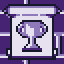Completed the Chambers
Beat all Chambers stagesBeat the Game
Finish the game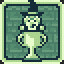Completed the Game
Beat all stages of the game

That’s all we are sharing today in Warlock’s Tower 100% Walkthrough and Achievement Guide , if there are anything you want to add please feel free to leave a comment below.